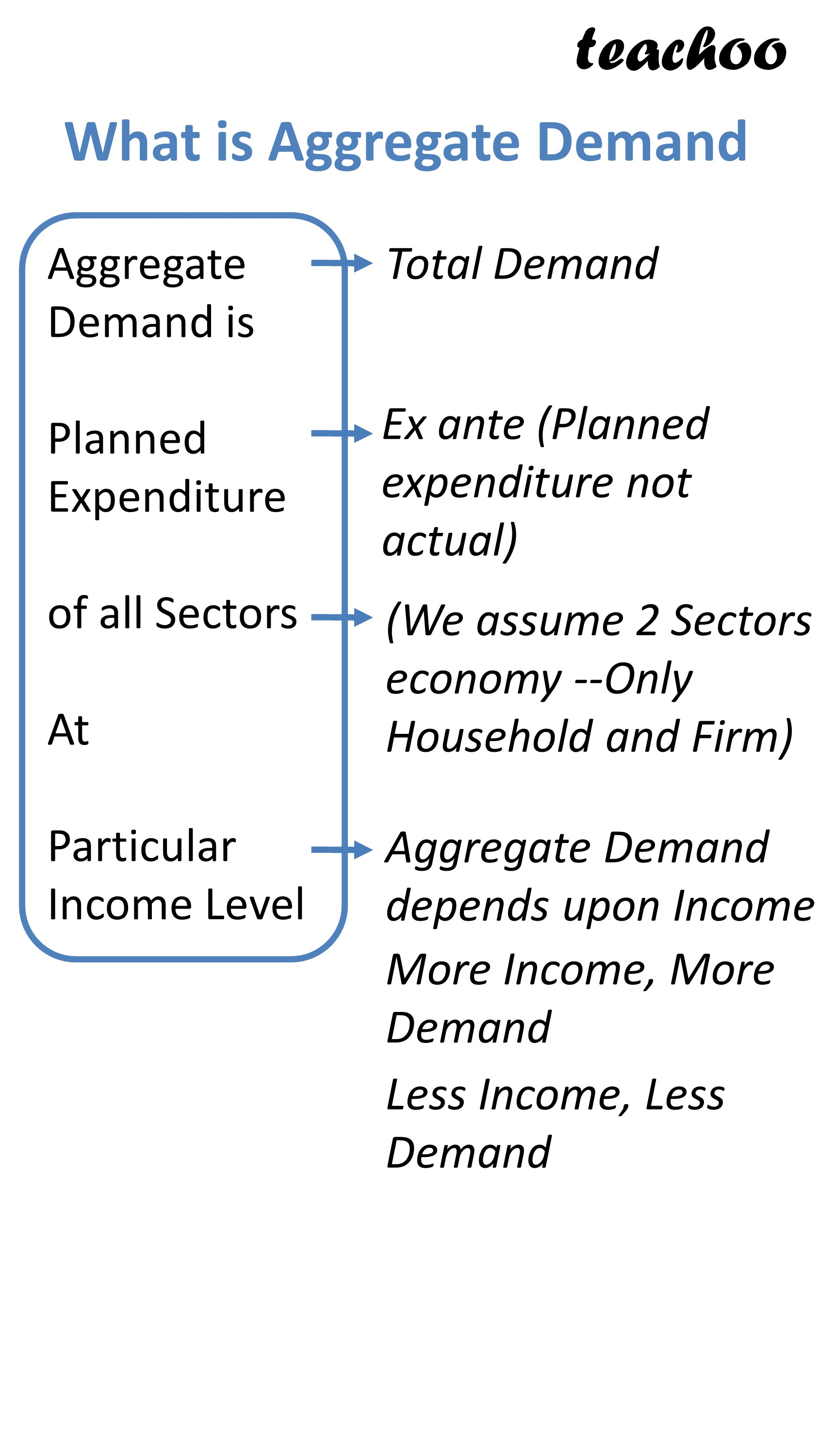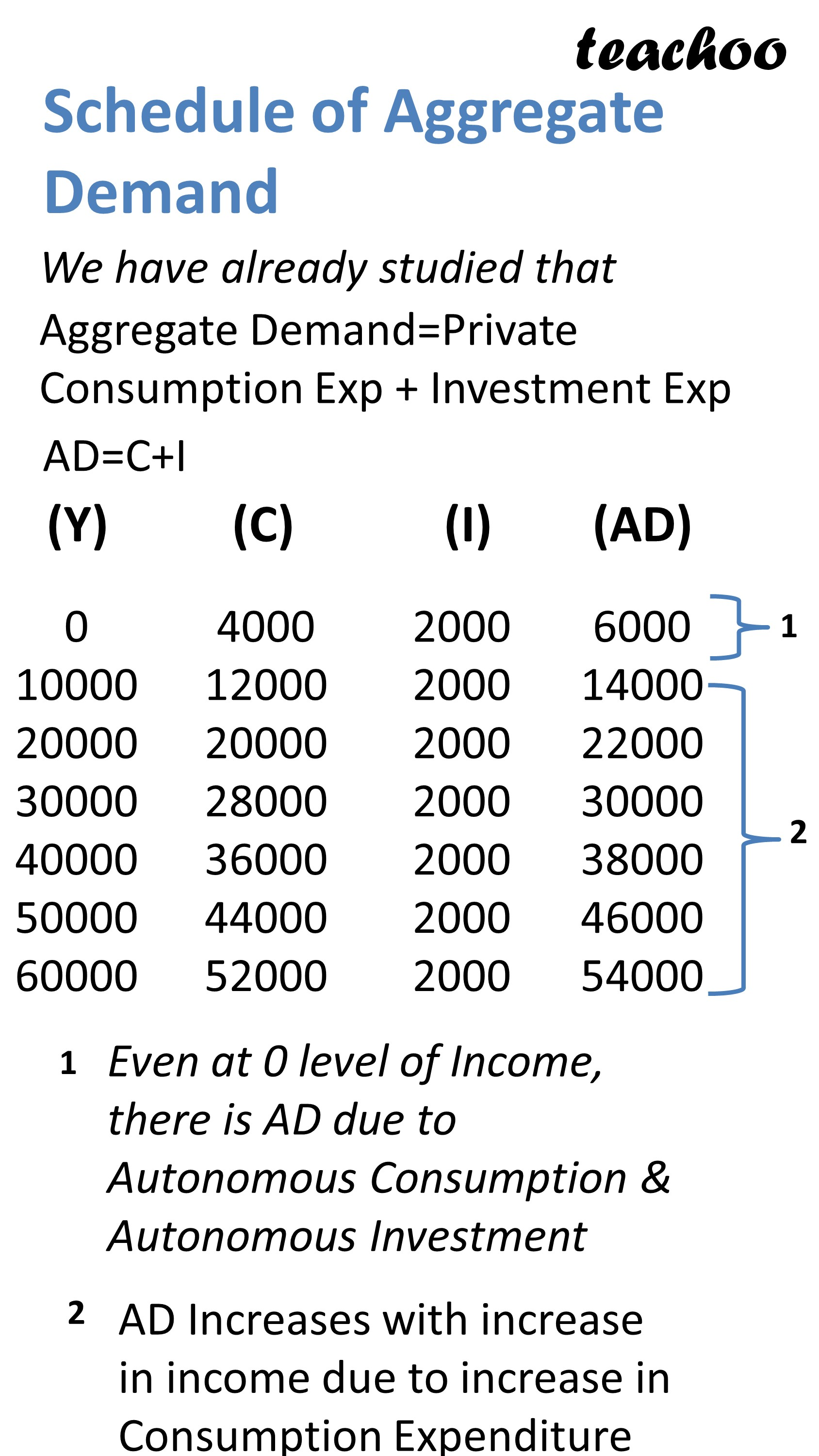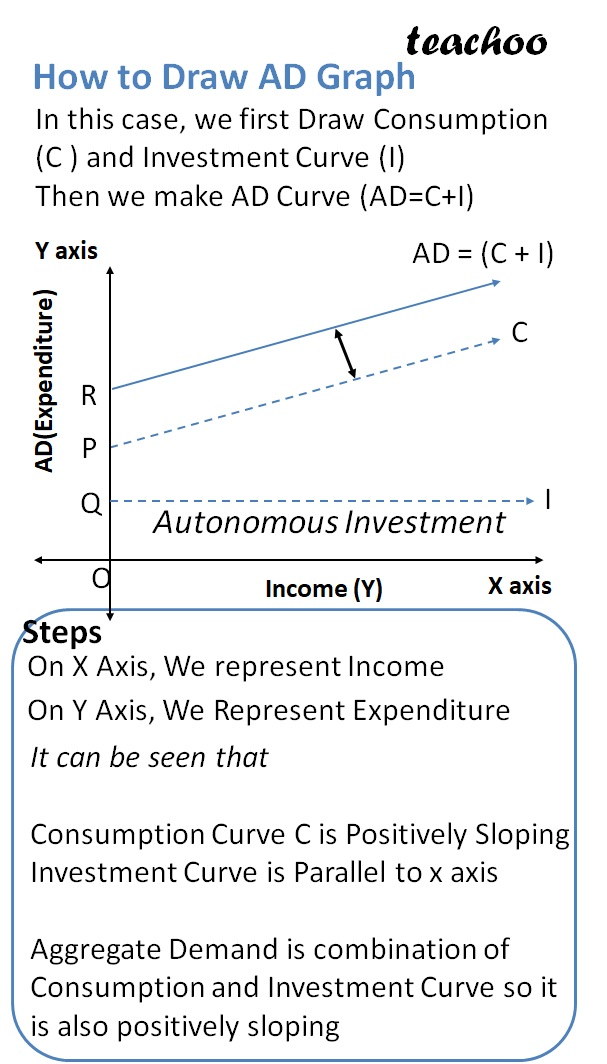Chapter 4 Part 1 - AD,AS and Related Concepts

Economics Class 12
Macroeconomics

## What is Aggregate Demand?

Aggregate Demand is planned expenditure of all sectors at Particular Income LevelAggregate Demand = Private Consumption Exp + Investment Expenditure

## Schedule of Aggregate Demand

It can be made by totaling Consumption and Investment Expenditure1. Even at 0 level of Income, there is AD

due to Autonomous Consumption and Autonomous Investment Expenditure which are applicable at 0 level of income

With the increase in income, Consumption Investment increases but investment expenditure remains the same

Due to this increase in consumption expenditure, AD increases with income

3. Note all 3 curves do not start from Origin

Consumption Curve starts from Point S (OS Representing Autonomous Consumption Expenditure)

Investment Curve starts from Point R (OR Represents Autonomous Investment Expenditure)

AD Curve starts from Point T (OT Represents total of Autonomous Consumption and Investment Expenditure)

OT = OR + OS

4. Consumption Curve C is Positively Sloping

Increases with Increase in Income

Investment Expenditure is a horizontal line parallel to x axis

It Remains Fixed irrespective of increase in income

Aggregate Demand is combination of Consumption and Investment Curve so it is also positively sloping

### NCERT Questions

No questions in this part

### Other Books

#### Question 1

State some important points about Aggregate Demand.

Learn in your speed, with individual attention - Teachoo Maths 1-on-1 Class

### Transcript

What is Aggregate Demand Aggregate Demand is Planned Expenditure of all Sectors At Particular Income Level Total Demand Ex ante (Planned expenditure not actual) (We assume 2 Sectors economy --Only Household and Firm) Aggregate Demand depends upon Income More Income, More Demand Less Income, Less Demand Schedule of Aggregate Demand We have already studied that Aggregate Demand=Private Consumption Exp + Investment Exp AD=C+I 0 10000 20000 30000 40000 50000 60000 4000 12000 20000 28000 36000 44000 52000 2000 2000 2000 2000 2000 2000 2000 6000 14000 22000 30000 38000 46000 54000 Even at 0 level of Income, there is AD due to Autonomous Consumption & Autonomous Investment AD Increases with increase in income due to increase in Consumption Expenditure How to Draw AD Graph In this case, we first Draw Consumption (C ) and Investment Curve (I) Then we make AD Curve (AD=C+I) Steps On X Axis, We represent Income On Y Axis, We Represent Expenditure It can be seen that Consumption Curve C is Positively Sloping Investment Curve is Parallel to x axis Aggregate Demand is combination of Consumption and Investment Curve so it is also positively sloping